Courses

# Systems Of Linear Equations, Matrix Algebra And Transform Theory - MCQ Test 1

## 20 Questions MCQ Test Topicwise Question Bank for GATE Civil Engineering | Systems Of Linear Equations, Matrix Algebra And Transform Theory - MCQ Test 1

Description
This mock test of Systems Of Linear Equations, Matrix Algebra And Transform Theory - MCQ Test 1 for Civil Engineering (CE) helps you for every Civil Engineering (CE) entrance exam. This contains 20 Multiple Choice Questions for Civil Engineering (CE) Systems Of Linear Equations, Matrix Algebra And Transform Theory - MCQ Test 1 (mcq) to study with solutions a complete question bank. The solved questions answers in this Systems Of Linear Equations, Matrix Algebra And Transform Theory - MCQ Test 1 quiz give you a good mix of easy questions and tough questions. Civil Engineering (CE) students definitely take this Systems Of Linear Equations, Matrix Algebra And Transform Theory - MCQ Test 1 exercise for a better result in the exam. You can find other Systems Of Linear Equations, Matrix Algebra And Transform Theory - MCQ Test 1 extra questions, long questions & short questions for Civil Engineering (CE) on EduRev as well by searching above.
QUESTION: 1

### The system of linear equations 4x + 2y = 7 2x + y = 6              has

Solution:

(b) This can be written as AX = B Where A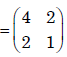Angemented matrix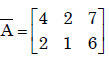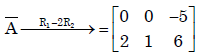rank(A) ≠ rank(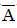). The system is inconsistant .So system has no solution.

QUESTION: 2

### For the following set of simultaneous equations: 1.5x – 0.5y = 2 4x + 2y + 3z = 9 7x + y + 5z = 10

Solution:

(a)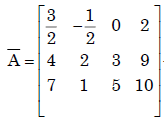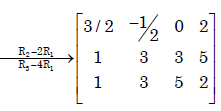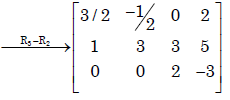∴ rank of() = rank of(A) = 3

∴ The system has unique solution.

QUESTION: 3

### The following set of equations has 3 x + 2 y + z = 4 x – y + z = 2 ​-2 x + 2 z = 5

Solution:

(b)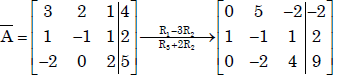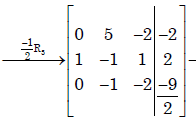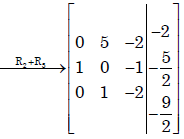∴ rank (A) = rank () = 3
∴ The system has unique solution

QUESTION: 4

Consider the system of simultaneous equations
x + 2y + z = 6
2x + y + 2z =  6
x + y +  z = 5
This system has

Solution:

(c )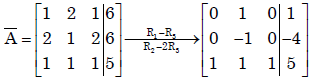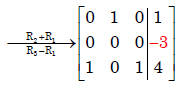∴ rank(A) = 2 ≠ 3 = rank() .

∴ The system is inconsistent and has no solution.

QUESTION: 5

Multiplication of matrices E and F is G. Matrices E and G are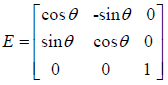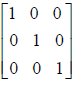What is the matrix F?

Solution:

(c)
Given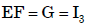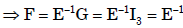QUESTION: 6

Consider a non-homogeneous system of linear equations representing mathematically an over-determined system. Such a system will be

Solution:

Ans.(b)
In an over determined system having more equations than variables, it is necessary to have consistent having many solutions .

QUESTION: 7

For the set of equations
x1 + 2x  + x3 + 4x4 = 0
3x1 + 6x2 + 3x3 + 12x4 = 0

Solution:

given set of equations are x1+2x2+x3+4x4=2 , 3x1+6x2+3x3+12x4=6

consider AB =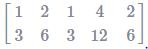⇒ R2 → R2 - 3R1

AB =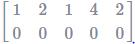P(A)=1; P(AB)=1;n=4

⇒ P(A) =P(B) < no. of variables

⇒ Infinitely many solutions ⇒multiple non-trivial solution

QUESTION: 8

Let P ≠ 0 be a 3 × 3 real matrix. There exist linearly independent vectors x and y such that Px = 0 and Py = 0. The dimension of the range space of P is

Solution:

(b)

QUESTION: 9

The eigen values of a skew-symmetric matrix are

Solution:

(c)

QUESTION: 10

The rank of a 3×3 matrix C (=AB), found by multiplying a non-zero column matrix Aof size 3×1 and a non-zero row matrix B of size 1×3, is

Solution:

(b)

Let A =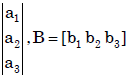Then C = AB =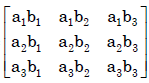Then det (AB) = 0.

Then also every minor
of order 2 is also zero.
∴ rank(C) =1.

QUESTION: 11

Match the items in columns I and II.
​Column I                                               Column II
P. Singular matrix                               1. Determinant is not defined
Q. Non-square matrix                          2. Determinant is always one
R. Real symmetric                              3. Determinant is zero
S. Orthogonal matrix                           4. Eigenvalues are always real
5. Eigenvalues are not defined

Solution:

(a) (P) Singular matrix → Determinant is zero
(Q) Non-square matrix → Determinant is not defined
(R) Real symmetric → Eigen values are always real
(S) Orthogonal → Determinant is always one

QUESTION: 12

Real matrices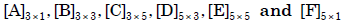are given. Matrices [B] and
[E] are symmetric.
Following statements are made with respect to these matrices.
1. Matrix product [F]T [C]T [B] [C] [F] is a scalar.
2. Matrix product [D]T [F] [D] is always symmetric.
With reference to above statements, which of the following applies?

Solution:

(a)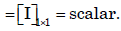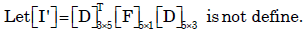QUESTION: 13

The product of matrices (PQ)–1 P is

Solution:

(b)
(PQ) -1 = P Q-1P-1P = Q-1

QUESTION: 14

The matrix A
=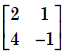is decomposed into a product of a lower triangular matrix [L] and an upper triangular matrix [U]. The properly decomposed [L] and [U] matrices respectively are

Solution:

Ans. (d)

QUESTION: 15

The inverse of the matrix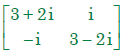is

Solution:

(b)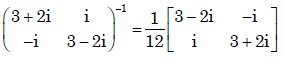QUESTION: 16

The inverse of the 2 × 2 matrix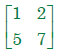is,

Solution:

(a).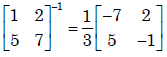QUESTION: 17

There are two containers, with one containing 4 Red and 3 Green balls and the other containing 3 Blue and 4 Green balls. One ball is drawn at random form each container.The probability that one of the ball is Red and the other is Blue will be

Solution: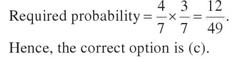QUESTION: 18

The Fourier transform of x(t) = e–at u(–t), where u(t) is the unit step function,

Solution:

Ans. (d)

QUESTION: 19

Given that F(s) is the one-sided Laplace transform of f(t), the Laplace transform of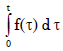is [EC:

Solution:

Ans. (b)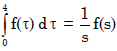……(Lapalace formule)

QUESTION: 20

If f(t) is a finite and continuous function for t, the Laplace transformation is given by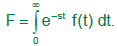For f(t) = cos h mt, the Laplace transformation is…..

Solution: